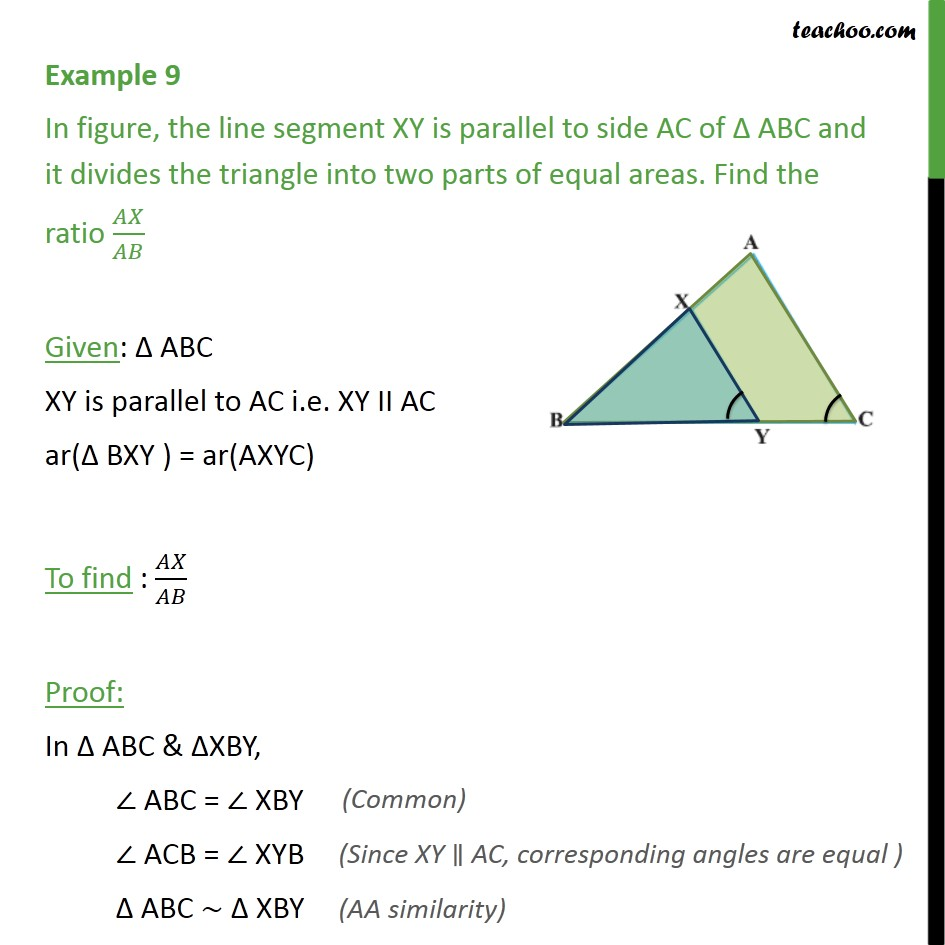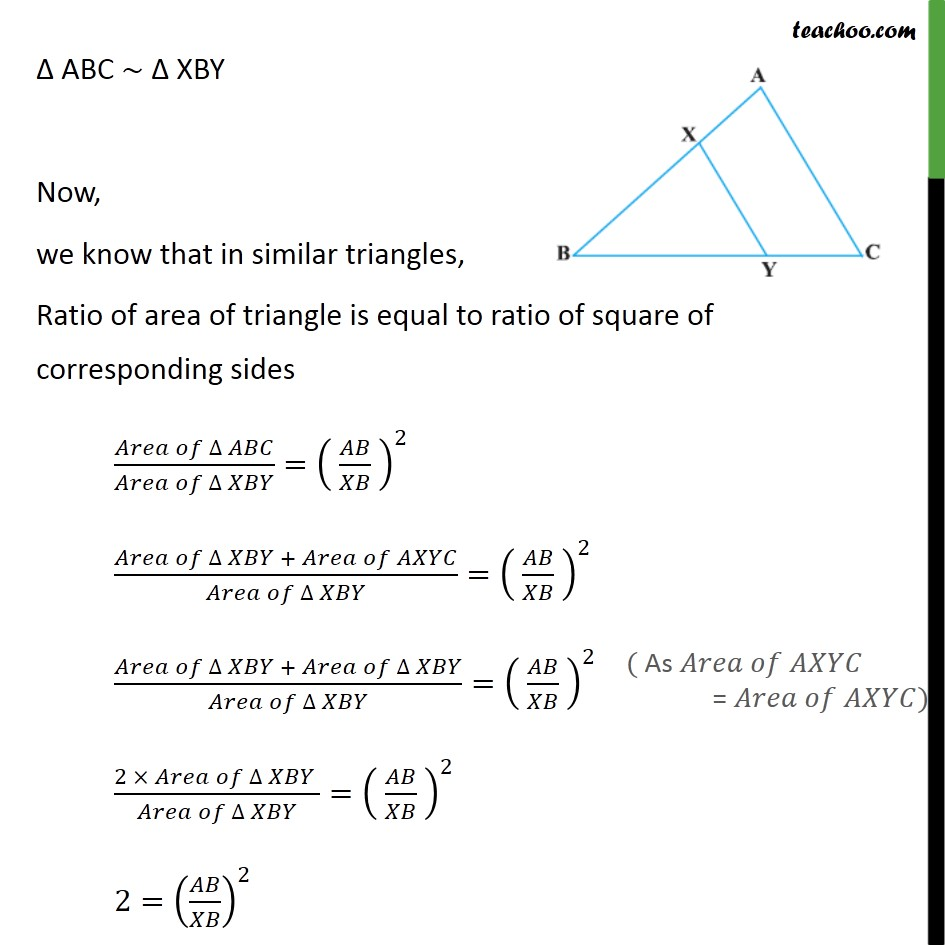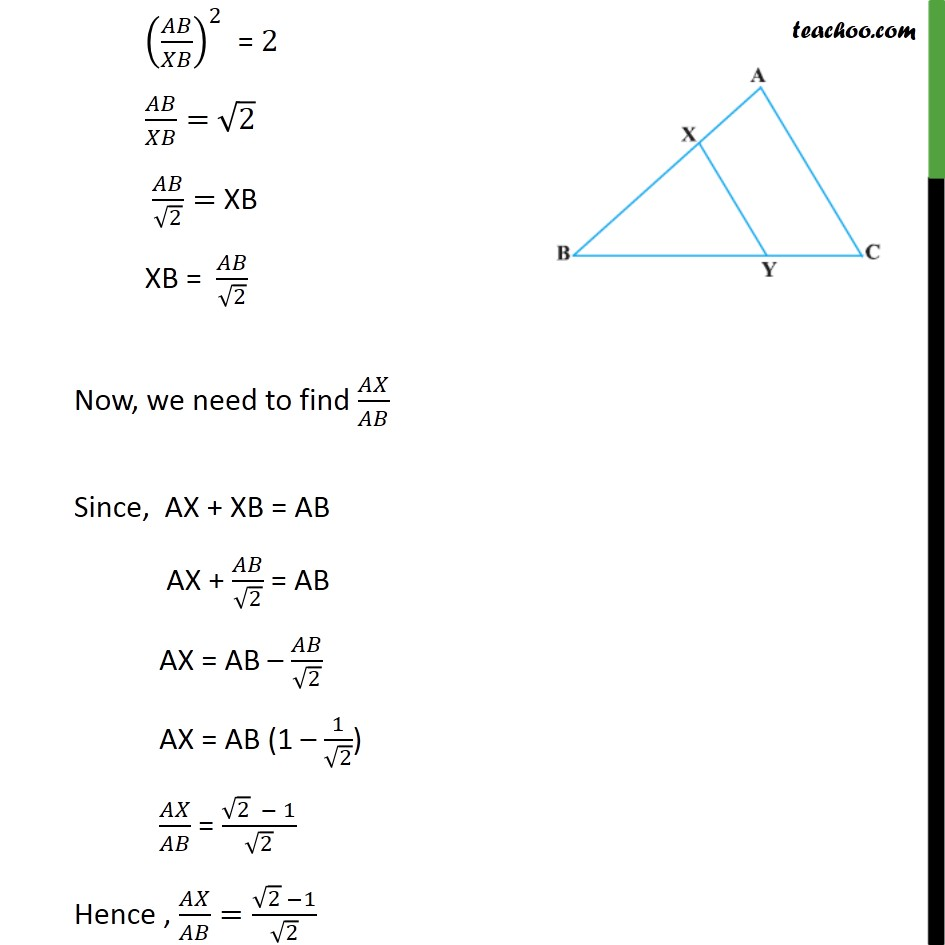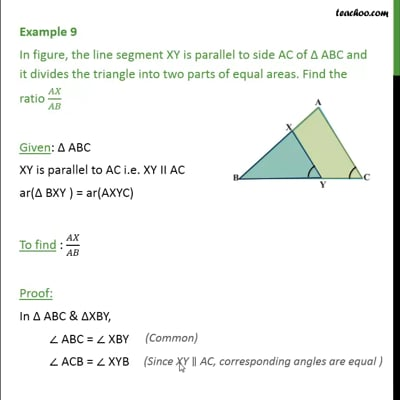Examples

Chapter 6 Class 10 Triangles
Serial order wiseThis video is only available for Teachoo black users

Solve all your doubts with Teachoo Black (new monthly pack available now!)

### Transcript

Example 9 In figure, the line segment XY is parallel to side AC of ABC and it divides the triangle into two parts of equal areas. Find the ratio / Given: ABC XY is parallel to AC i.e. XY II AC ar( BXY ) = ar(AXYC) To find : / Proof: In ABC & XBY, ABC = XBY ACB = XYB ABC ~ XBY ABC ~ XBY Now, we know that in similar triangles, Ratio of area of triangle is equal to ratio of square of corresponding sides ( )/( )=( / )^2 ( + )/( )=( / )^2 ( + )/( )=( / )^2 (2 )/( )=( / )^2 2=( / )^2 ( / )^2 = 2 / = 2 / 2= XB XB = / 2 Now, we need to find / Since, AX + XB = AB AX + / 2 = AB AX = AB / 2 AX = AB (1 1/ 2) / = ( 2 1)/ 2 Hence , / =( 2 1)/ 2